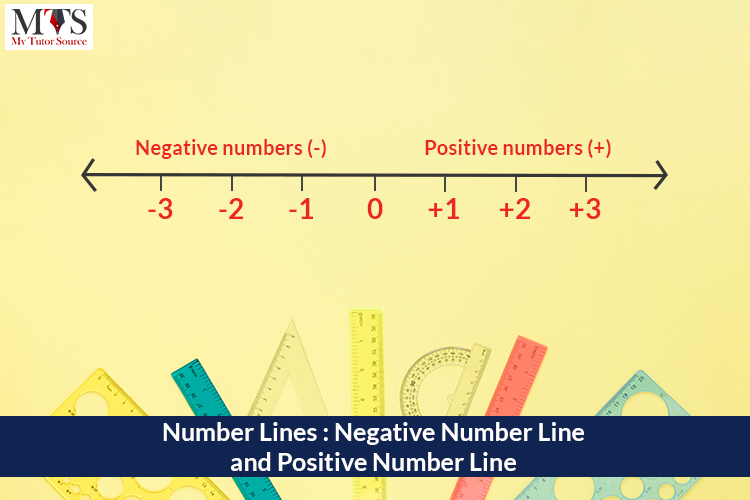# Number Lines: Negative Number Line and Positive Number LineIn mathematics, a horizontal straight line with numbers or integers placed at equal segments or intervals along its length is known as the Number Line. It starts from zero, and the numbers increase when you move from zero to the right and decrease from zero to the left. Shifting to right or left indicates the negative or positive directions.

The number line is used for addition and subtraction purposes in mathematics. It has two sides, a Negative Number Line and Positive Number Line. Both sides can be extended infinitely in a horizontal manner. Also, the ends of number lines are indicated by arrows.

## Negative Number Line

The left side of a number line is called a negative number line. It increases in number but with a negative sign, and the negative sign means the value of the number or integer is decreasing. The left side of the number lines is used for subtraction and addition purposes mostly.

Numbers on the left side of zero are always smaller than the numbers on the right side.

### Example:

To add numbers on the negative number line, you must know how to use addition and subtraction signs. For adding (-1) and (-4), look for -1 number on the negative number lines and move 4 steps in the left direction. The number you will get after moving four steps forward will be the answer.

## Positive Number Line

The right side of a number line is called the positive number line. It increases in numbers too, but they all are whole numbers. The right side of the number lines is used for the addition and multiplication of numbers mostly.

### Example:

To add (1) and (7) on a number line. Look for number 1 and then move 7 steps forward in the right direction, drawing a line in the horizontal direction. The number you will get after moving seven steps from point 1 will be the answer.

## Usage of Number Line

Number lines with negative numbers and positive numbers are used to teach children the basic concepts of addition and subtraction in Mathematics. It is also used for multiplication by skipping count. Solving equations of negative numbers of a number line is tricky as positive or negative signs are used.

Apparently, number lines seem like an essential method of teaching kids the concepts of addition, subtraction, and multiplication, but they can be used in numerous other ways. People use the negative number line and positive number lines to calculate and measure details. These lines show the significant increase and decrease of values.

Brush up on your mathematical skills and attain the highest grades with our experienced math tutors. Take a free trial now.

### Find Top Tutors in Your AreaWith over 3 years of experience in teaching, Chloe is very deeply connected with the topics that talk about the educational and general aspects of a student's life. Her writing has been very helpful for students to gain a better understanding of their academics and personal well-being. I’m also open to any suggestions that you might have! Please reach out to me at chloedaniel402 [at] gmail.com